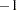The ROBUSTREG Procedure
 OUTEST= Data Set

The OUTEST= data set contains parameter estimates for the model. You can specify a label in the MODEL statement to distinguish between the estimates for different models used by the ROBUSTREG procedure. If the COVOUT option is specified, the OUTEST= data set also contains the estimated covariance matrix of the parameter estimates. Note that, if the ROBUSTREG procedure does not converge, the parameter estimates are set to missing in the OUTEST data set.

The OUTEST= data set contains all variables specified in the MODEL statement and the BY statement. One observation consists of parameter values for the model with the dependent variable having the value. If the COVOUT option is specified, there are additional observations containing the rows of the estimated covariance matrix. For these observations, the dependent variable contains the parameter estimate for the corresponding row variable. The following variables are also added to the data set:

_MODEL_

is a character variable containing the label of the MODEL statement, if present. Otherwise, the variable’s value is blank.

_NAME_

is a character variable containing the name of the dependent variable for the parameter estimates or the name of the row for the covariance matrix estimates.

_TYPE_

is a character variable containing the type of the observation, either PARMS for parameter estimates or COV for covariance estimates.

_METHOD_

is a character variable containing the type of estimation method, either M estimation, LTS estimation, S estimation, or MM estimation.

_STATUS_

is a character variable containing the status of model fitting, either Converged, Warning, or Failed.

INTERCEPT

is a numeric variable containing the intercept parameter estimates and covariances.

_SCALE_

is a numeric variable containing the scale parameter estimates.

Any BY variables specified are also added to the OUTEST= data set.Previous Page | Next Page | Top of Page